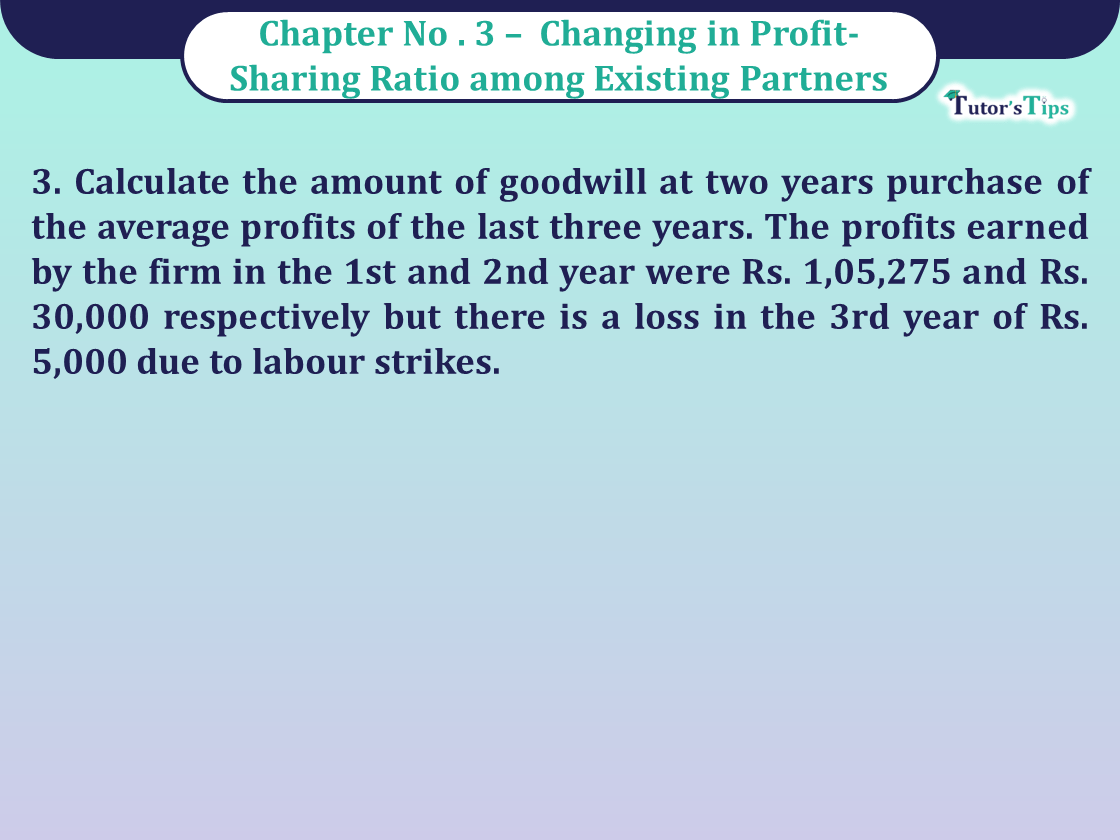# Question 3 Chapter 3 of Class 12 Part – 1 VK PublicationQuestion 3 Chapter 3 of Class 12 Part - 1 VK Publication

Question 3 Chapter 3 of Class 12 Part – 1

3. Calculate the amount of goodwill at two years purchase of the average profits of the last three years. The profits earned by the firm in the 1st and 2nd year were Rs. 1,05,275 and Rs. 30,000 respectively but there is a loss in the 3rd year of Rs. 5,000 due to labour strikes.

## The solution of Question 3 Chapter 3 of Class 12 Part – 1: –

 Average Profit = Total Profit for past given years Number of years = 1,05,275+30,000-5,000 3 = 1,30,275 3 = 43,425

Goodwill = Average Profit x Number of Years’ Purchase = 43,425 x 2 = Rs. 86,8500.

Comment if you have any questions.

Also, Check out the solved question of previous Chapters: –